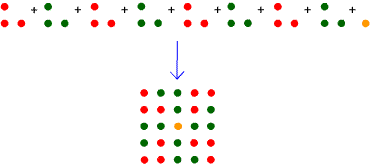#### You may also like### Odd Differences

The diagram illustrates the formula: 1 + 3 + 5 + ... + (2n - 1) = nÂ² Use the diagram to show that any odd number is the difference of two squares.### Triangular Triples

Show that 8778, 10296 and 13530 are three triangular numbers and that they form a Pythagorean triple.# Triangles Within Squares

##### Age 14 to 16Challenge LevelThe diagram above shows that: $$8 \times T_2 + 1 = 25 = 5^2$$

Use a similar method to help you verify that: $$8 \times T_3 + 1 = 49 = 7^2$$ Can you generalise this result?

Can you find a rule in terms of $T_n$ and a related square number?

Can you find a similar rule involving square numbers for $T_{n}, T_{n+2}$ and several copies of $T_{n+1}$?